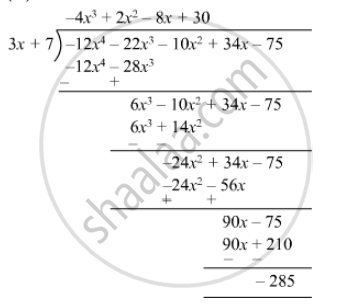Advertisement Remove all ads

# Verify the Division Algorithm I.E. Dividend = Divisor × Quotient + Remainder, in Each of the Following. Also, Write the Quotient and Remainder. Dividend Divisor 34x − 22x3 − 12x4 − 10x2 − 75 3x + 7 - Mathematics

Sum

Verify the division algorithm i.e. Dividend = Divisor × Quotient + Remainder, in each of the following. Also, write the quotient and remainder.

 Dividend Divisor 34x − 22x3 − 12x4 − 10x2 − 75 3x + 7
Advertisement Remove all ads

#### SolutionQuotient  = - 4x3 + 2x2 - 8x + 30

Remainder  = - 285

Divisor  = 3x + 7

Divisor x  Quotient + Remainder =  (3x + 7) (-  4x3 + 2x2 - 8x + 30) - 285

=  12x4 + 6x3 - 24x2 + 90x - 28x3 + 14x2 - 56x + 210 - 285

= - 12x 4 - 22x3 -10x2 + 34x - 75

=  Dividend

Thus,

Divisor x  Quotient + Remainder = Dividend

Hence verified.

Is there an error in this question or solution?
Advertisement Remove all ads

#### APPEARS IN

RD Sharma Class 8 Maths
Chapter 8 Division of Algebraic Expressions
Exercise 8.4 | Q 21.4 | Page 12
Advertisement Remove all ads

#### Video TutorialsVIEW ALL 

Advertisement Remove all ads
Share
Notifications

View all notifications

Forgot password?
Course